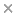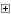BaseSpace
Correlation Engine 2.0Search sequence regions

###### filter terms:
• asians (1)
• cancer (7)
• ethnic groups (1)
• humans (1)
• IL 1 (3)
• Il 1β (11)
• meta analysis (2)
• odds ratio (23)
• public health (1)
• rs3783553 (2)
• Sizes of these terms reflect their relevance to your search.

Colorectal cancer (CRC) is a major public health problem given its high incidence and mortality. This study focuses on examining the associations between IL-1α, IL-1β, and IL-1RN polymorphisms and colorectal cancer susceptibility. A systematic literature search of PubMed, Embase, Web of Science, CNKI (China National Knowledge Infrastructure) and Wan Fang databases was conducted to identify relevant studies. Relevant data were extracted from the original included studies. The correlation was demonstrated based on the odds ratio (OR) and corresponding 95% confidence intervals (95% CIs). Publication bias was investigated by Egger's line regression test and Begg's funnel plot. Eighteen independent studies involving 6218 cases and 10160 controls were eligible for this pooled analysis. Overall, the result revealed that the IL-1α rs3783553 polymorphism was significantly associated with an increased risk of CRC (G vs. C, OR = 1.02, 95% CI = 0.90-1.15, I2 = 51%, P = 0.78; GG vs. CC, OR = 1.97, 95% CI = 1.04-3.74, I2 = 70%, P = 0.04; GC vs. CC, OR = 1.75, 95% CI = 1.12-2.75, I2 = 42%, P = 0.01; GG + GC vs. CC, OR = 1.85, 95% CI = 1.08-3.18, I2 = 63%, P = 0.03; and GG vs. GC + CC, OR = 1.28, 95% CI = 1.04-1.58, I2 = 39%, P = 0.02), and significance was also noted for IL-1RN VNTR under the dominant model (22 + 2L vs. LL, OR = 1.49, 95% CI = 1.01-2.19, I2 = 77%, P = 0.045) and allelic contrast model (2 vs. L, OR = 1.28, 95% CI = 1.00-1.64, I2 = 58.6%, P = 0.047). For IL-1β + 31C/T, significance was observed in the dominant model (CC + CT vs. TT, OR = 0.83, 95% CI = 0.69-0.99, I2 = 52%, P = 0.034) and the heterozygous model (CT vs. TT, OR = 0.80, 95% CI = 0.65-0.98, I2 = 60%, P = 0.04). For IL-1β + 511 C/T, a significant association was noted in four gene models (CT vs. TT, OR = 0.72, 95% CI = 0.63-0.83, I2 = 0%, P < 0.001; CC + CT vs. TT, OR = 0.74, 95% CI = 0.65-0.84, I2 = 0%, P < 0.001; CC vs. TT, OR = 0.77, 95% CI = 0.65-0.91, I2 = 30.9%, P = 0.003; C vs. T, OR = 0.87, 95% CI = 0.80-0.95, I2 = 38%, P = 0.001), but a significant relationship was not found in the recessive model (CC vs. CT + TT, OR = 1.09, 95% CI = 0.86-1.38, I2 = 57.1%, P = 0.25). In addition, borderline statistical significance was noted between IL-1β + 3954 Ins/Del and CRC in the homozygous model, but no significance was identified for IL-1β + 3737 G/A, Il-1β + 1464 G/C, and IL-1RN + 2018 T/C under all five genetic models. In the subgroup analysis of ethnic groups, significant associations with CRC were found for IL-1β + 31 (CC vs. TT: OR = 0.82, 95% CI = 0.67-0.99, I2 = 20.2%, P = 0.04; CT vs. TT: OR = 0.62, 95% CI = 0.47-0.82, I2 = 0%, P < 0.001; CC + CT vs. TT: OR = 0.69, 95% CI = 0.55-0.87, I2 = 29.7%, P = 0.001), IL-1β + 511 (CT vs. TT, OR = 0.65, 95% CI = 0.55-0.77, I2 = 0%, P < 0.001; CC + CT vs. TT, OR = 0.67, 95% CI = 0.58-0.78, I2 = 0%, P < 0.001; C vs. T, OR = 0.83, 95% CI = 0.75-0.92, I2 = 49.6%, P < 0.001) and IL-1RN + 2018 T/C in the allelic contrast model (T vs. C, OR = 0.66, 95% CI = 0.44-0.98, I2 = 0%, P = 0.04) among Asians but not in Caucasians. A significant association between IL-1β + 1464 G/C polymorphisms in Caucasians was observed under the recessive model (OR = 0.87, 95% CI = 0.77-0.98, I2 = 45%, P = 0.03). The current meta-analysis demonstrated that IL-1α rs3783553, IL-1β + 31C/T, IL-1β + 511C/T, and IL-1RN VNTR are critical genes for CRC susceptibility. Copyright © 2020 Elsevier B.V. All rights reserved.

### Citation

Li Liu, Zhenglong Zhai, Danyang Wang, Yun Ding, Xiaoqing Chen, Qiqi Wang, Zheyue Shu, Minglan Wu, Lei Chen, Xuelin He, Dazhi Fan, Faming Pan, Meiyuan Xing. The association between IL-1 family gene polymorphisms and colorectal cancer: A meta-analysis. Gene. 2021 Feb 15;769:145187

### var substancesSectionCollapsed = true;Substances

PMID: 32998046

View Full Text